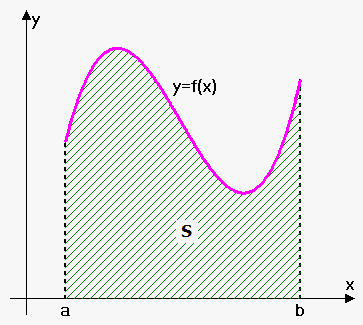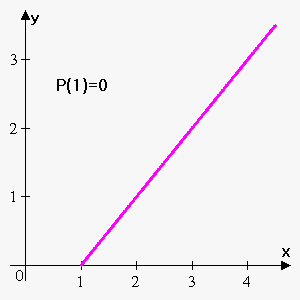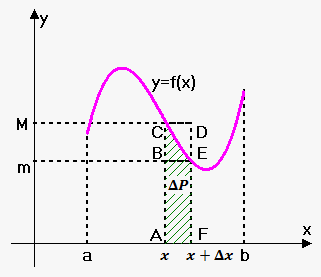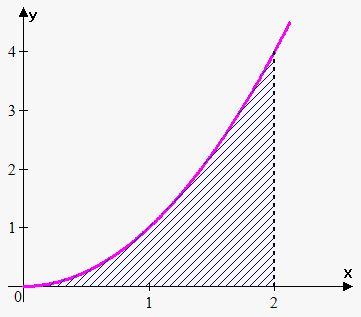Area Problem

Related calculator: Definite and Improper Integral Calculator

Suppose that we are given continuous function $y={f{{\left({x}\right)}}}$ on ${\left[{a},{b}\right]}$ such that ${f{{\left({x}\right)}}}\ge{0}$ for all${x}\in{\left[{a},{b}\right]}$.

We want to find area ${S}$ that lies under curve ${f{{\left({x}\right)}}}$ and bounded by lines ${x}={a}$, ${x}={b}$ and x-axis.

Before doing this imagine right bound ${b}$ is not fixed, we can move it. Then for different values of right bound we will obtain different values of area, so we can consider area as function ${P}{\left({x}\right)}$ of right bound. In particular, ${P}{\left({a}\right)}={0}$ (because area from ${a}$ to ${a}$ is 0) and ${P}{\left({b}\right)}={S}$.

To get a better understanding what is ${P}{\left({x}\right)}$ consider next example.

Example 1. Consider function ${y}={x}-{1}$. If ${P}{\left({x}\right)}$ represents area of figure under ${y}={x}$, above x-axis on interval ${\left[{1},{x}\right]}$ find ${P}{\left({1}\right)}$, ${P}{\left({2}\right)}$, ${P}{\left({3}\right)}$ and ${P}{\left({4}\right)}$.${P}{\left({1}\right)}$ is area on interval ${\left[{1},{1}\right]}$, so it is 0 (because length of interval is 0).

${P}{\left({2}\right)}$ is area on interval ${\left[{1},{2}\right]}$. It is area of right-angled triangle with legs 1 and 1. So, ${P}{\left({2}\right)}=\frac{{1}}{{2}}\cdot{1}\cdot{1}=\frac{{1}}{{2}}$.

${P}{\left({3}\right)}$ is area on interval ${\left[{1},{3}\right]}$. It is area of right-angled triangle with legs 2 and 2. So, ${P}{\left({3}\right)}=\frac{{1}}{{2}}\cdot{2}\cdot{2}={2}$.

${P}{\left({4}\right)}$ is area on interval ${\left[{1},{4}\right]}$. It is area of right-angled triangle with legs 3 and 3. So, ${P}{\left({4}\right)}=\frac{{1}}{{2}}\cdot{3}\cdot{3}=\frac{{9}}{{2}}$.

As can be seen ${P}{\left({x}\right)}$ is indeed function: for any particular value of ${x}$ there is corresponding ${P}{\left({x}\right)}$.Now, suppose we want to find derivative of ${P}{\left({x}\right)}$. For this increase ${x}$ by $\Delta{x}$. Corresponding increase in area is $\Delta{P}={P}{\left({x}+\Delta{x}\right)}-{P}{\left({x}\right)}$ (figure ACEF).

If we denote maximum and minimum value of ${f{{\left({x}\right)}}}$ on interval ${\left[{x},{x}+\Delta{x}\right]}$ by ${M}$ and ${m}$ respectively, then area of figure ACEF is less than area of rectangle ACDF and greater than area of rectangle ABEF.

Since area of rectangle ACDF is ${M}\Delta{x}$ (height multiplied by base)and area of rectangle ABEF is ${m}\Delta{x}$ then

${m}\Delta{x}<\Delta{P}<{M}\Delta{x}$ or ${m}<\frac{{\Delta{P}}}{{\Delta{x}}}<{M}$.

Now let $\Delta{x}\to{0}$. Since ${f{}}$ is continuous then both ${m}$ and ${M}$ approach ${f{{\left({x}\right)}}}$.

Thus, by Squeeze Theorem $\lim_{{\Delta{x}\to{0}}}\frac{{\Delta{P}}}{{\Delta{x}}}={f{{\left({x}\right)}}}$.

But we recognize in $\lim_{{\Delta{x}\to{0}}}\frac{{\Delta{P}}}{{\Delta{x}}}$ derivative of ${P}{\left({x}\right)}$, so ${P}'{\left({x}\right)}={f{{\left({x}\right)}}}$.

In other words ${P}{\left({x}\right)}=\int{f{{\left({x}\right)}}}{d}{x}={F}{\left({x}\right)}+{C}$.

Since ${P}{\left({a}\right)}={0}$ then ${0}={P}{\left({a}\right)}={F}{\left({a}\right)}+{C}$ or ${C}=-{F}{\left({a}\right)}$.

Therefore, ${P}{\left({x}\right)}={F}{\left({x}\right)}-{F}{\left({a}\right)}$.

Newton-Leibniz Formula. Area of figure ${P}{\left({x}\right)}$ that lies under curve ${y}={f{{\left({x}\right)}}}$, above x-axis on interval ${\left[{a},{x}\right]}$ is ${P}{\left({x}\right)}={F}{\left({x}\right)}-{F}{\left({a}\right)}$.

Returning to our initial problem we have that ${S}={F}{\left({b}\right)}-{F}{\left({a}\right)}$.

Area of figure that lies under curve ${y}={f{{\left({x}\right)}}}$, above x-axis and between lines ${x}={a}$ and ${x}={b}$ is ${S}={F}{\left({b}\right)}-{F}{\left({a}\right)}$ where ${F}$ is antiderivative of ${f{}}$.

Example 2. Find area under ${y}={{x}}^{{2}}$, above x-axis on interval ${\left[{0},{2}\right]}$.We have that ${F}{\left({x}\right)}=\int{{x}}^{{2}}{d}{x}=\frac{{1}}{{3}}{{x}}^{{3}}$ (note that we need particular derivative here).

Now, according to Newton-Leibniz Formula required area is ${S}={F}{\left({2}\right)}-{F}{\left({0}\right)}=\frac{{1}}{{3}}\cdot{{2}}^{{3}}-\frac{{1}}{{3}}\cdot{{0}}^{{3}}=\frac{{8}}{{3}}$.

All from above can be easily extended on case when function can take negative values. For this we only need to consider areas under x-axis negative.

Indeed, if we want to find area enclose by parabola ${y}=-{{x}}^{{2}}$, x-axis on interval ${\left[{0},{2}\right]}$ then according to Newton-Leibniz Formula we have that ${S}={F}{\left({2}\right)}-{F}{\left({0}\right)}$ where ${F}{\left({x}\right)}=\int-{{x}}^{{2}}{d}{x}=-\frac{{1}}{{3}}{{x}}^{{3}}$. So, ${S}=-\frac{{8}}{{3}}$. Since $-{{x}}^{{2}}$ is reflection of ${{x}}^{{2}}$ about x-axis then this area lies below x-axis, and, therefore, negative.